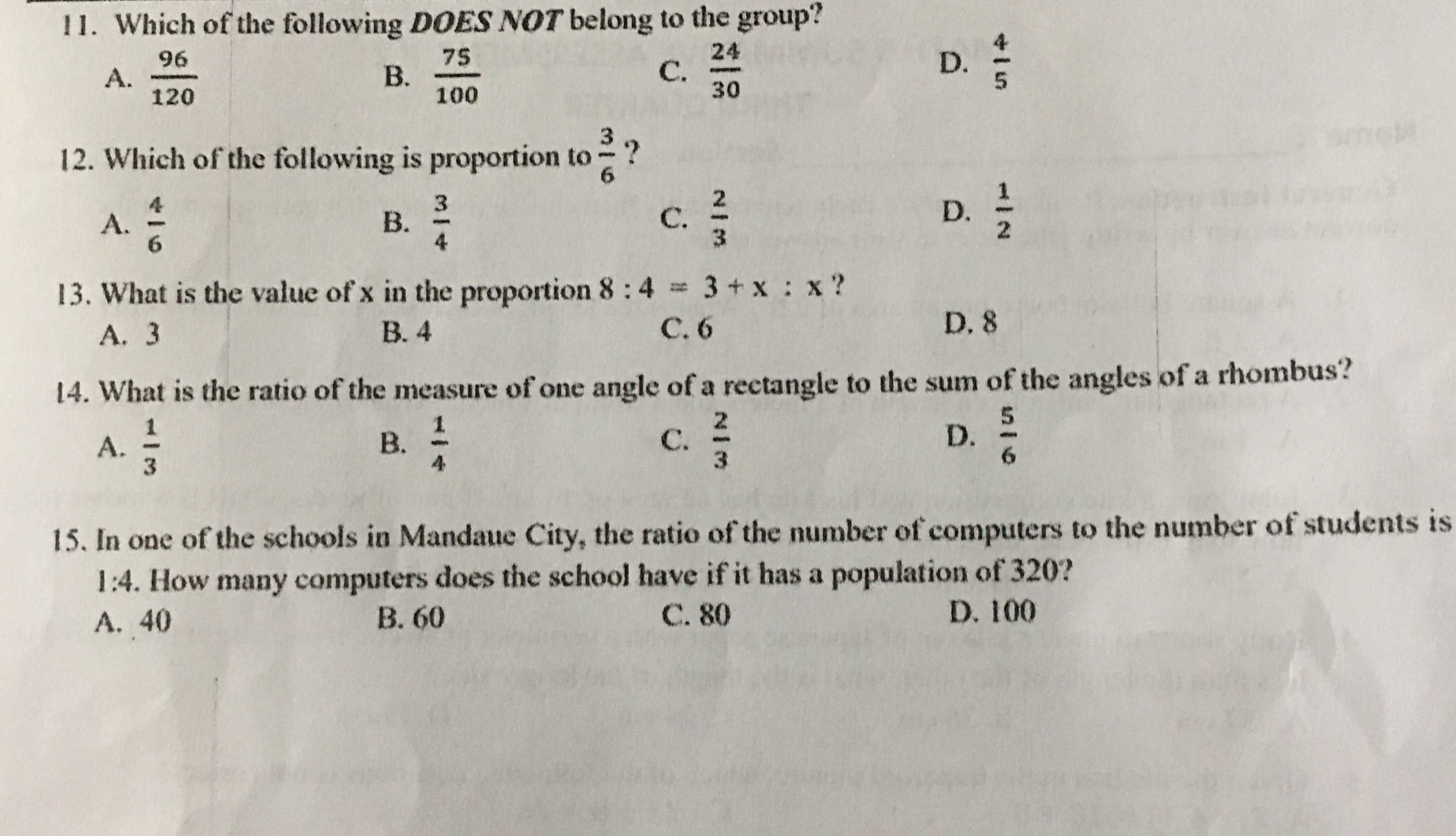### ¿Todavía tienes preguntas de matemáticas?

Pregunte a nuestros tutores expertos
Algebra
Pregunta11. Which of the following DOES NOT belong to the group?

A. $$\frac { 96 } { 120 }$$ B. $$\frac { 75 } { 100 }$$ C. $$\frac { 24 } { 30 }$$ D. $$\frac { 4 } { 5 }$$

12. Which of the following is proportion to $$\frac { 2 } { 6 }$$ ?

A. $$\frac { 4 } { 6 }$$ B. $$\frac { 3 } { 4 }$$ C. $$\frac { 2 } { 3 }$$ D. $$\frac { 1 } { 2 }$$

13. What is the value of $$x$$ in the proportion $$8 : 4 = 3 + x : x$$ ?

A. $$3$$ B. $$4$$ C. $$6$$ D. $$8$$

14. What is the ratio of the measure of one angle of a rectangle to the sum of the angles of a rhombus?

A. $$\frac { 1 } { 3 }$$ B. $$\frac { 1 } { 4 }$$ C. $$\frac { 2 } { 3 }$$ D. $$\frac { 5 } { 6 }$$

15. In one of the schools in Mandaue City, the ratio of the number of computers to the number of students is 1:4. How many computers does the school have if it has a population of $$320$$ ?

A. $$40$$ B. $$60$$ C. $$80$$ D. $$100$$